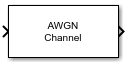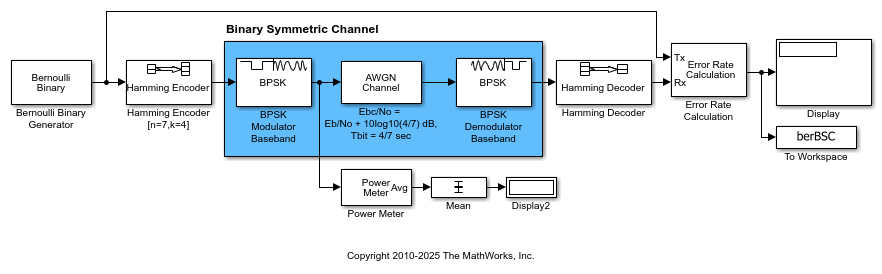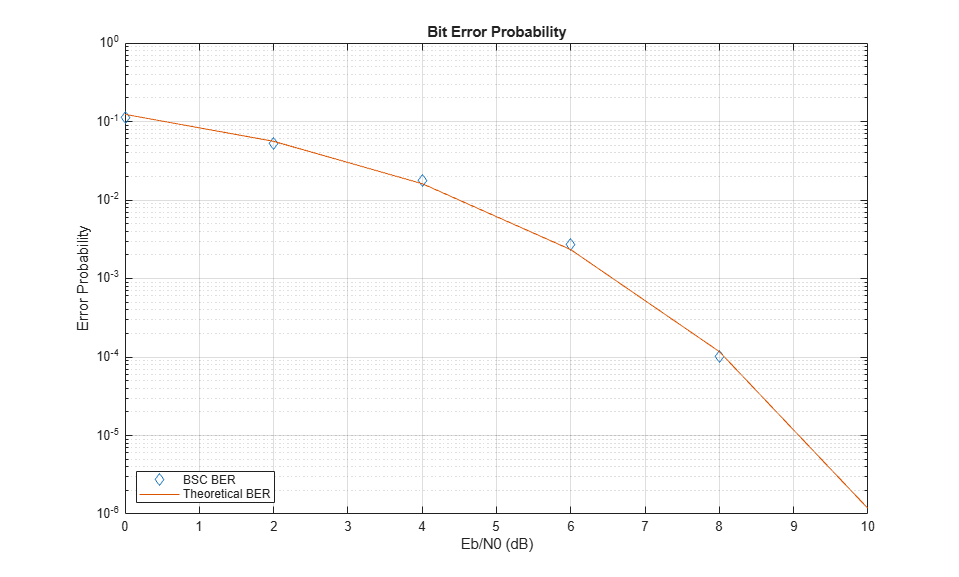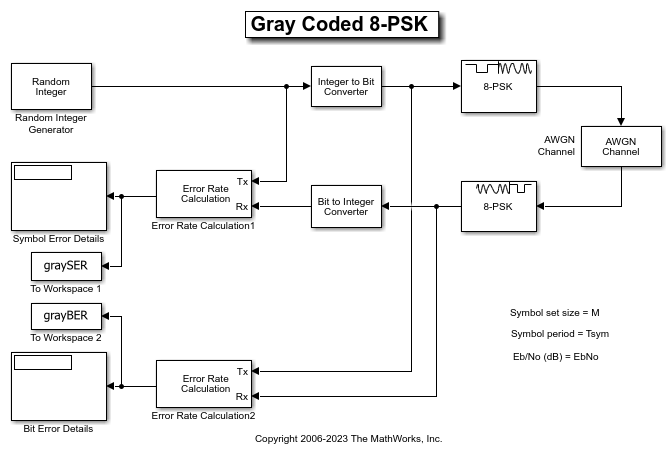# AWGN Channel

Add white Gaussian noise to input signal

•Libraries:
Communications Toolbox / Channels

## Description

The AWGN Channel block adds white Gaussian noise to the input signal. It inherits the sample time from the input signal.

## Examples

expand all

When your simulation uses coding, the Eb/N0 setting for the AWGN channel must be adjusted by the coding rate to apply the desired signal to noise (SNR) ratio.

The slex_hamming_check.slx model performs forward error control (FEC) coding on a BPSK-modulated signal that gets filtered through an AWGN channel. The model uses BPSK Modulator Baseband, AWGN Channel, and BPSK Demodulator Baseband discrete blocks to simulate a binary symmetric channel. To account for difference between the coded and uncoded Eb/N0 (Eb/N0), the AWGN channel block computes the coded Eb/N0 as Eb/N0 + 10log10(K/N) dB, where K/N is the code rate and Eb/N0 is the uncoded Eb/N0. The Hamming Encoder block has an input bit period of 1 second and the output bit period decreases, by a factor of the K/N code rate, to 4/7 seconds. Due to the coding rate, the Binary Symmetric Channel has a bit period of 4/7 seconds.

The model initializes variables used to configure block parameters by using the PreLoadFcn callback function. For more information, see Model Callbacks (Simulink).

model = 'slex_hamming_check'; open_system(model);The AWGN channel block must also configure the Number of bits per symbol and Input signal power, referenced to 1 ohm (watts) parameter settings based on the modulated signal. This example uses BPSK modulation, so the AWGN Channel block has number of bits per symbol set to 1. The model includes a Power Meter block that measures the signal power at the AWGN input to confirm the setting required for the input signal power of the AWGN Channel block.

Produce error rate results and a plot to compare simulation with theory. The theoretical channel error probability for the coded signal is Q(sqrt(2*Ebc/N0)), where Q() is the standard Q function and Ebc/N0 is the coded Eb/N0 in linear units (not in dB). Compute the theoretical BER upper limit bound of a linear, rate 4/7 block code with a minimum distance of 3, and hard decision decoding for a BPSK-modulated signal in AWGN over a range of Eb/N0 values by using the bercoding function. Simulate the slex_hamming_check model over the same range of Eb/N0 values.

EbNoVec = 0:2:10; theorBER = bercoding(EbNoVec,'block','hard',7,4,3); berVecBSC = zeros(length(EbNoVec),3); for n = 1:length(EbNoVec) EbNo = EbNoVec(n); sim(model); berVecBSC(n,:) = berBSC(end,1); end 

Plot the results by using the semilogy function to show the nearly identical results. The model has the Error Rate Calculation block configured to run each Eb/N0 point until 200 errors occur or the block receives 1x10^6 bits.

semilogy( ... EbNoVec,berVecBSC(:,1),'d', ... EbNoVec,theorBER,'-'); legend('BSC BER','Theoretical BER', ... Location="southwest"); xlabel("Eb/N0 (dB)"); ylabel("Error Probability"); title("Bit Error Probability"); grid on;This model shows the improvement in BER performance when using log-likelihood ratio (LLR) instead of hard decision demodulation in a convolutionally coded communication link.

For a MATLAB® version of this example, see Log-Likelihood Ratio (LLR) Demodulation.

System Setup

This example model simulates a convolutionally coded communication system having one transmitter, an AWGN channel and three receivers. The convolutional encoder has a code rate of 1/2. The system employs a 16-QAM modulation. The modulated signal passes through an additive white Gaussian noise channel. The top receiver performs hard decision demodulation in conjunction with a Viterbi decoder that is set up to perform hard decision decoding. The second receiver has the demodulator configured to compute log-likelihood ratios (LLRs) that are then quantized using a 3-bit quantizer. It is well known that the quantization levels are dependent on noise variance for optimum performance . The exact boundaries of the quantizer are empirically determined here. A Viterbi decoder that is set up for soft decision decoding processes these quantized values. The LLR values computed by the demodulator are multiplied by -1 to map them to the right quantizer index for use with Viterbi Decoder. To compute the LLR, the demodulator must be given the variance of noise as seen at its input. The third receiver includes a demodulator that computes LLRs which are processed by a Viterbi decoder that is set up in unquantized mode. The BER performance of each receiver is computed and displayed.

modelName = 'commLLRvsHD'; open_system(modelName);System Simulation and Visualization

Simulate this system over a range of information bit Eb/No values. Adjust these Eb/No values for coded bits and multi-bit symbols to get noise variance values required for the AWGN block and Rectangular QAM Baseband Demodulator block. Collect BER results for each Eb/No value and visualize the results.

EbNo = 2:0.5:8; % information rate Eb/No in dB codeRate = 1/2; % code rate of convolutional encoder nBits = 4; % number of bits in a 16-QAM symbol Pavg = 10; % average signal power of a 16-QAM modulated signal snr = EbNo - 10*log10(1/codeRate) + 10*log10(nBits); % SNR in dB noiseVarVector = Pavg ./ (10.^(snr./10)); % noise variance % Initialize variables for storing the BER results ber_HD = zeros(1,length(EbNo)); ber_SD = zeros(1,length(EbNo)); ber_LLR = zeros(1, length(EbNo)); % Loop over all noiseVarVector values for idx=1:length(noiseVarVector) noiseVar = noiseVarVector(idx); %#ok<NASGU> sim(modelName); % Collect BER results ber_HD(idx) = BER_HD(1); ber_SD(idx) = BER_SD(1); ber_LLR(idx) = BER_LLR(1); end % Perform curve fitting and plot the results fitBER_HD = real(berfit(EbNo,ber_HD)); fitBER_SD = real(berfit(EbNo,ber_SD)); fitBER_LLR = real(berfit(EbNo,ber_LLR)); semilogy(EbNo,ber_HD,'r*', ... EbNo,ber_SD,'g*', ... EbNo,ber_LLR,'b*', ... EbNo,fitBER_HD,'r', ... EbNo,fitBER_SD,'g', ... EbNo,fitBER_LLR,'b'); legend('Hard Decision Decoding', ... 'Soft Decision Decoding','Unquantized Decoding'); xlabel('Eb/No (dB)'); ylabel('BER'); title('LLR vs. Hard Decision Demodulation with Viterbi Decoding'); grid on;To experiment with this system further, try different modulation types. This system uses a binary mapped modulation scheme for faster error collection but it is well known that Gray mapped signal constellation provides better BER performance. Experiment with various constellation ordering options in the modulator and demodulator blocks. Configure the demodulator block to compute approximate LLR to see the difference in the BER performance compared to hard decision demodulation and LLR. Try out a different range of Eb/No values. Finally, investigate different quantizer boundaries for your modulation scheme and Eb/No values.

You can configure this example to use data-driven execution by setting the Domain parameter to dataflow for Dataflow Subsystem. With dataflow, blocks inside the domain, execute based on the availability of data as rather than the sample timing in Simulink®. Simulink automatically partitions the system into concurrent threads. This autopartitioning accelerates simulation and increases data throughput. To learn more about dataflow and how to run this example using multiple threads, see Multicore Simulation of Comparing Demodulation Types.

% Cleanup close_system(modelName,0); clear modelName EbNo codeRate nBits Pavg snr noiseVarVector ... ber_HD ber_SD ber_LLR idx noiseVar fitBER_HD fitBER_SD fitBER_LLR; 

Selected Bibliography

 J. L. Massey, "Coding and Modulation in Digital Communications", Proc. Int. Zurich Seminar on Digital Communications, 1974

 J. A. Heller, I. M. Jacobs, "Viterbi Decoding for Satellite and Space Communication", IEEE® Trans. Comm. Tech. vol COM-19, October 1971

This example uses the doc_gray_code to compute bit error rates (BER) and symbol error rates (SER) for M-PSK modulation. The theoretical error rate performance of M-PSK modulation in AWGN is compared to the error rate performance for Gray-coded symbol mapping and to the error rate performance of binary-coded symbol mapping.The Random Integer Generator block serves as the source, producing a sequence of integers. The Integer to Bit Converter block converts each integer into a corresponding binary representation. The M-PSK Modulator Baseband block in the doc_gray_code model:

• Accepts binary-valued inputs that represent integers in the range [0, (M - 1], where M is the modulation order.

• Maps binary representations to constellation points using a Gray-coded ordering.

• Produces unit-magnitude complex phasor outputs, with evenly spaced phases in the range [0, (2(M - 1) / M)].

The AWGN Channel block adds white Gaussian noise to the modulated data. The M-PSK Demodulator Baseband block demodulates the noisy data. The Bit to Integer Converter block converts each binary representation to a corresponding integer. Then two separate Error Rate Calculation blocks calculate the error rates of the demodulated data. The block labeled SER Calculation compares the integer data to compute the symbol error rate statistics and the block labeled BER Calculation compares the bits data to compute the bit error rate statistics. The output of the Error Rate Calculation block is a three-element vector containing the calculated error rate, the number of errors observed, and the amount of data processed.

To reduce simulation run time and ensure that the statistics of the errors remain stable as the Eb/N0 ratio increases, the model is configured to run until 100 errors occur or until 1e8 bits have been transmitted.

The model initializes variables used to configure block parameters by using the PreLoadFcn callback function. For more information, see Model Callbacks (Simulink).

Produce Error Rate Curves

Compute the theoretical BER for nondifferential 8-PSK in AWGN over a range of Eb/N0 values by using the berawgn function. Simulate the doc_gray_code model with Gray-coded symbol mapping over the same range of Eb/N0 values.

Compare Gray coding with binary coding, by modifying the M-PSK Modulator Baseband and M-PSK Demodulator Baseband blocks to set the Constellation ordering parameter to Binary instead of Gray. Simulate the doc_gray_code model with binary-coded symbol mapping over the same range of Eb/N0 values.

Plot the results by using the semilogy function. The Gray-coded system achieves better error rate performance than the binary-coded system. Further, the Gray-coded error rate aligns with the theoretical error rate statistics.## Ports

### Input

expand all

Input data signal, specified as an NS-by-1 vector or an NS-by-NC matrix. NS represents the number of samples in the input signal. NC represents the number of channels, as determined by the number of columns in the input signal matrix. Both NS and NC can be equal to 1.

The block adds frames of length-NS Gaussian noise to each of the NC channels, using a distinct random distribution per channel.

Data Types: double | single
Complex Number Support: Yes

Variance of additive white Gaussian noise, specified as a positive scalar or a 1-by-NC vector. NC represents the number of channels, as determined by the number of columns in the input signal matrix. For more information, see Specifying the Variance Directly or Indirectly.

#### Dependencies

To enable this port, set Mode to Variance and Noise variance source to Input port.

Data Types: double

### Output

expand all

Output data signal for the AWGN channel, returned as a vector or matrix. The datatype and dimensions of Out match those of the input signal, In.

## Parameters

expand all

To edit block parameters interactively, use the Property Inspector. From the Simulink® Toolstrip, on the Simulation tab, in the Prepare gallery, select .

Variance mode, specified as Signal to noise ratio (Eb/No), Signal to noise ratio (Es/No), Signal to noise ratio (SNR), or Variance. For more information, see Relationship Among Eb/No, Es/No, and SNR Modes and Specifying the Variance Directly or Indirectly.

Ratio of signal power to noise power in decibels, specified as a scalar or vector.

Tunable: Yes

#### Dependencies

To enable this parameter, set Mode to Signal to noise ratio (SNR).

Ratio of information bit energy per symbol to noise power spectral density in decibels, specified as a scalar or vector. The information bit energy is the magnitude without channel coding.

Tunable: Yes

#### Dependencies

To enable this parameter, set Mode to Signal to noise ratio (Eb/No).

Ratio of information symbol energy per symbol to noise power spectral density in decibels, specified as a scalar or vector. The information symbol energy is the magnitude without channel coding.

Tunable: Yes

#### Dependencies

To enable this parameter, set Mode to Signal to noise ratio (Es/No).

Number of bits in each input symbol, specified as a scalar or vector.

#### Dependencies

To enable this parameter, set Mode to Signal to noise ratio (Eb/No).

Mean square power of the input in watts, specified as a scalar or vector.

• When Mode is Signal to noise ratio (Eb/No) or Signal to noise ratio (Es/No), the parameter is the mean square power of the input symbols.

• When Mode is Signal to noise ratio (SNR), this parameter is the mean square power of the input samples.

Tunable: Yes

#### Dependencies

To enable this parameter, set Mode to Signal to noise ratio (Eb/No), Signal to noise ratio (Es/No), or Signal to noise ratio (SNR).

Samples per symbol in samples, specified as a positive scalar or vector.

#### Dependencies

To enable this parameter, set Mode to Signal to noise ratio (Eb/No) or Signal to noise ratio (Es/No).

Noise variance source, specified as a Parameter or Input port. For more information, see Specifying the Variance Directly or Indirectly.

Tunable: Yes

#### Dependencies

To enable this parameter, set Mode to Variance.

Variance of the white Gaussian noise, specified as a scalar or vector. For more information, see Specifying the Variance Directly or Indirectly.

Tunable: Yes

#### Dependencies

To enable this port, set Mode to Variance and Noise variance source to Parameter.

Randomization

Random number source, specified as a Global stream or mt19937ar with seed.

Tunable: Yes

Noise generator initial seed for the mt19937ar algorithm, specified as a positive scalar or a 1-by-NC vector.

When the input signal is complex, the block creates random data as:

randData = randn(2*NS,NC) noise = randData(1:2:end) + 1i(randData(2:2:end))
NS is the number of samples and NC is the number of channels.

You can specify different seed values for each DLL build.

Tunable: Yes

#### Dependencies

To enable this parameter, set Random number source to mt19937ar with seed.

Type of simulation to run, specified as Code generation or Interpreted execution.

• Code generation — Simulate the model by using generated C code. The first time you run a simulation, Simulink generates C code for the block. The model reuses the C code for subsequent simulations unless the model changes. This option requires additional startup time, but the speed of the subsequent simulations is faster than with the Interpreted execution option.

• Interpreted execution — Simulate the model by using the MATLAB® interpreter. This option shortens startup time, but the speed of subsequent simulations is slower than with the Code generation option. In this mode, you can debug the source code of the block.

## Block Characteristics

 Data Types double | single Multidimensional Signals no Variable-Size Signals yes

## Tips

• You can tune parameters in normal mode, accelerator mode, or rapid accelerator mode.

• Unless otherwise indicated, parameters are nontunable.

• For nontunable parameters, when you use the Simulink Coder™ rapid simulation (RSIM) target to build an RSIM executable, you cannot change their values without recompiling the model.

• If a parameter is tunable, you can change its value at any time. This is useful for Monte Carlo simulations in which you run the simulation multiple times (such as on multiple computers) with different amounts of noise.

## Algorithms

expand all

 Proakis, John G. Digital Communications. 4th Ed. McGraw-Hill, 2001.